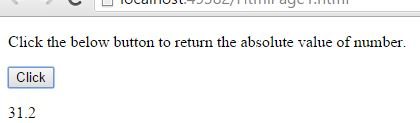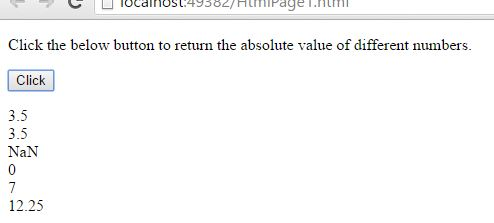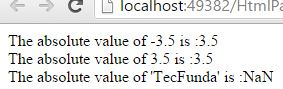# abs() (Absolute value) in JavaScript

##### How to return the absolute value of a number or different numbers in JavaScript?

By using `abs()` method we can return the absolute value of a number or different numbers.

The `abs()` method is used to return the absolute value of a number in JavaScript, the `abs()` method is a static method of math, so we always use it as `Math.abs()`, here in this case `Math` is not a constructor.

### Returning the absolute value of a number

```<p>Click the below button to return the absolute value of number.</p>
<input type="button" onclick="myNumber()" value="Click">
<p id="myId"></p>

<script>
function myNumber() {
document.getElementById("myId").innerHTML = Math.abs(-31.2);
}
</script>
```

In the above code snippet we have given `Id` as "`myId`"to the second `<p>` element in the HTML code. There is a function myNumber() in the`<script>`block which is connected to the onclick of the HTML button. We need to return the absolute value of a number, for that we are using `abs()` method. Onclick of the button "Click" in the HTML code fires the function myNuber() in the `<script>` block at the same time `Math.abs(-31.2)` method returns the absolute value of a given input number as output.

OUTPUT### Returning the absolute values of different numbers. (Basic way for returning the values)

```<p>Click the below button to return the absolute value of different numbers.</p>
<input type="button" onclick="myFunction()" value="Click">
<p id="myId"></p>

<script>
function myFunction() {
var a = Math.abs(3.5);
var b = Math.abs(-3.5);
var c = Math.abs("TechFunda");
var d = Math.abs(null);
var e = Math.abs(a + b);
var f = Math.abs(a * b);

var r = a + "<br>" + b + "<br>" + c + "<br>" + d + "<br>" + e + "<br>" + f;
document.getElementById("myId").innerHTML = r;
}
</script>
```

Absolute values of different numbers

Onclick of the button "Click" in the HTML code fires the function myNuber() in the `<script>` block at the same time `Math.abs()` method returns the absolute value of different input numbers as output.

OUTPUT### Absolute values

```<script>
var a = Math.abs(3.5);
document.write("The absolute value of -3.5 is :" + a);
var b = Math.abs(-3.5);
document.write("</br>The absolute value of 3.5 is :" + b);
var c = Math.abs("TechFunda");
document.write("</br>The absolute value of 'TecFunda' is :" + c);
</script>
```

Absolute values

This is the one of the way for returning the absolute values of different numbers by using `abs()` method. The output depends upon the given input numbers, but all the different ways of same input numbers gives the same output.

OUTPUTViews: 6561 | Post Order: 95